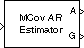Documentation

# Modified Covariance AR Estimator

Compute estimate of autoregressive (AR) model parameters using modified covariance method

## Library

Estimation / Parametric Estimation

`dspparest3`## Description

The Modified Covariance AR Estimator block uses the modified covariance method to fit an autoregressive (AR) model to the input data. This method minimizes the forward and backward prediction errors in the least squares sense. The input is a frame of consecutive time samples, which is assumed to be the output of an AR system driven by white noise. The block computes the normalized estimate of the AR system parameters, A(z), independently for each successive input.

`$H\left(z\right)=\frac{G}{A\left(z\right)}=\frac{G}{1+a\left(2\right){z}^{-1}+\dots +a\left(p+1\right){z}^{-p}}$`

You specify the order, p, of the all-pole model in the Estimation order parameter. To guarantee a valid output, you must set the Estimation order parameter to be less than or equal to two thirds the input vector length.

The output port labeled A outputs the normalized estimate of the AR model coefficients in descending powers of z.

`[1 a(2) ... a(p+1)] `

The scalar gain, G, is output from the output port labeled G.

See the Burg AR Estimator block reference page for a comparison of the Burg AR Estimator, Covariance AR Estimator, Modified Covariance AR Estimator, and Yule-Walker AR Estimator blocks.

## Parameters

Estimation order

Specify the order of the AR model, p.

## References

Kay, S. M. Modern Spectral Estimation: Theory and Application. Englewood Cliffs, NJ: Prentice-Hall, 1988.

Marple, S. L., Jr., Digital Spectral Analysis with Applications. Englewood Cliffs, NJ: Prentice-Hall, 1987.

## Supported Data Types

PortSupported Data Types

Input

• Double-precision floating point

• Single-precision floating point

A

• Double-precision floating point

• Single-precision floating point

G

• Double-precision floating point

• Single-precision floating point

The output data type is the same as the input data type.

 Burg AR Estimator DSP System Toolbox Covariance AR Estimator DSP System Toolbox Modified Covariance Method DSP System Toolbox Yule-Walker AR Estimator DSP System Toolbox `armcov` Signal Processing Toolbox